# Integral equation of convolution type

An integral equation containing the unknown function under the integral sign of a convolution transform (see Integral operator). The peculiarity of an integral equation of convolution type is that the kernel of such an equation depends on the difference of the arguments. The simplest example is the equation(1)

whereandare given functions andis the unknown function. Suppose thatand that one seeks a solution in the same class. In order that (1) is solvable it is necessary and sufficient that the following condition holds: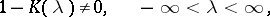(2)

whereis the Fourier transform of. When (2) holds, equation (1) has a unique solution in the class, representable by the formula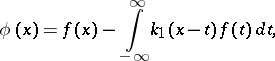(3)

where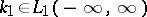is uniquely determined by its Fourier transform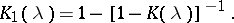An equation of convolution type on the half-line (a Wiener–Hopf equation)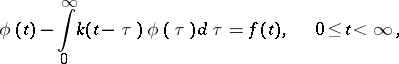(4)

arises in the study of various questions of theoretical and applied character (see , ).

Suppose that the right-hand sideand the unknown functionbelong to,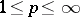, that the kernel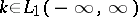and that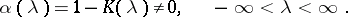(5)

The functionis called the symbol of equation (4). The index of equation (4) is the number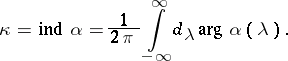(6)

If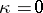, then the functions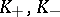defined by the equations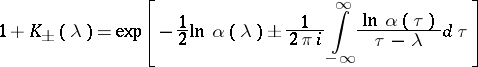(7)

are the Fourier transforms of functions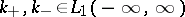, respectively, such thatfor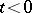. Under the above conditions, equation (4) has a unique solution, which can be expressed by the formula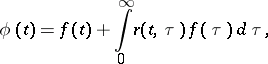(8)

where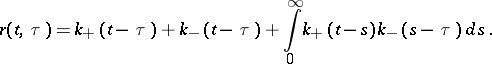If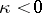, all solutions of equation (4) are given by the formula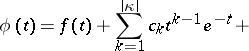(9)whereare arbitrary constants,(10)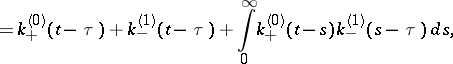and the functions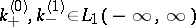are uniquely determined by their Fourier transforms: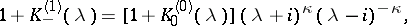(11)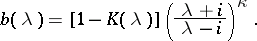When, the homogeneous equation corresponding to (4) has exactly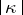linearly independent solutions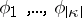, which are absolutely-continuous functions on any bounded interval; these solutions can be chosen so that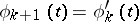,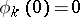for, and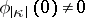.

If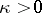, the equation is solvable only if the following condition holds: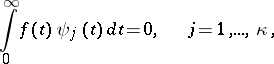(12)

whereis a system of linearly independent solutions of the transposed homogeneous equation of (4):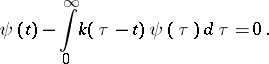(13)

Under these conditions, the (unique) solution is given by the formula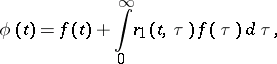where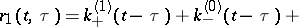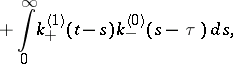while the Fourier transformsand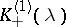of the functionsare defined by the equation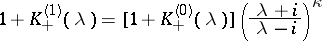and the equations (11). Noether's theorem holds for equation (4) (see Singular integral equation).

The first significant results in the theory of equations (4) were obtained in , where an effective method (the so-called Wiener–Hopf method) for solving the homogeneous equation corresponding to (4) was given under the hypothesis that the kernel and the required solution satisfy the conditions: For some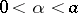bothThe main point of the Wiener–Hopf method is the idea of factorization of a function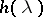which is holomorphic in the strip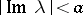, that is, the possibility to represent it as a product, where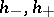are functions holomorphic in the half-planes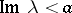and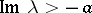, respectively, and satisfy certain additional requirements. These results have been developed and amplified (see ).

A method has been developed for reducing equation (4) to a boundary value problem of linear identification. In this way, equation (4) has been solved under the following hypotheses: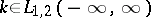,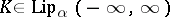,,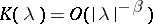,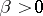, as, and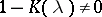,.

In addition to this, the role of the number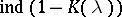in solving (4) has been clarified. In earlier articles an analogous role was played by the number of zeros of the analytic functionin a strip (see ).

Condition (5) is both necessary and sufficient in order that Noether's theorem holds for equation (4). The solution of equation (4) given above simplifies in a number of particular cases important in practice. The asymptotics of the solution have been obtained for special right-hand sides (see ).

Equation (4) has also been studied in the case whenand the Fourier transform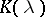of the kernel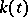has discontinuities of the first kind (see ) or is an almost-periodic function (see ). In these cases, condition (5) turns out to be insufficient for Noether's theorem to hold.

The validity of the majority of results listed above has also been established for systems of equations of type (4); however, in contrast to the case of a single equation, a system of integral equations of convolution type in the general case cannot be solved explicitly by quadratures (see ).

Also related to integral equations of convolution type are paired equations (or dual equations)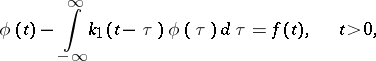(15)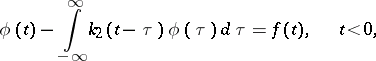and their transposed equations, so-called integral equations of convolution type with two kernels: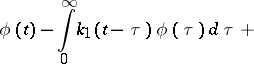(16)Equations (15) have been explicitly solved by quadratures in , and equation (16) in .

An integral equation of convolution type on a bounded interval,(17)

where, is called a Fredholm equation (see , ).

Integral equations of convolution type whose symbol vanishes at a finite number of points and the orders of whose zeros are integers, lend themselves to an explicit solution by quadratures (see , ).